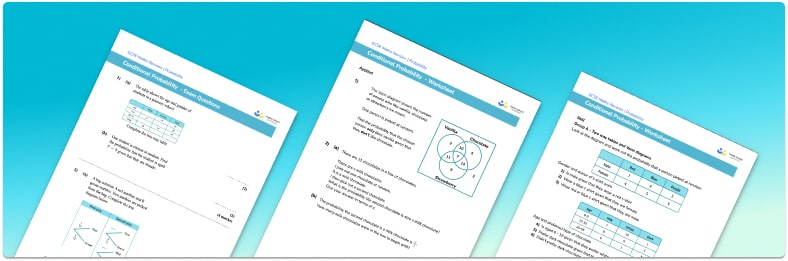# Conditional Probability Worksheet• Section 1 of the conditional probability worksheet contains 36 skills-based conditional probability questions, in 3 groups to support differentiation
• Section 2 contains 4 applied conditional probability questions with a mix of worded problems and deeper problem solving questions
• Section 3 contains 3 foundation and higher level GCSE exam style conditional probability questions
• Answers and a mark scheme for all conditional probability questions are provided
• Questions follow variation theory with plenty of opportunities for students to work independently at their own level
• All questions created by fully qualified expert secondary maths teachers

Suitable for GCSE maths revision for AQA, OCR and Edexcel exam boards

• This field is for validation purposes and should be left unchanged.

You can unsubscribe at any time (each email we send will contain an easy way to unsubscribe). To find out more about how we use your data, see our privacy policy.

### Conditional probability at a glance

Conditional probability is the probability of an event occurring given another event has already occurred. When finding probability based on a condition, we need to consider what has occurred before. For example, if we have a bag containing 3 red marbles and 2 blue marbles, the probability of picking a red marble is 0.6, and the probability of picking a blue marble is 0.2. If we pick one marble out then these values change. If a red marble is picked (and not replaced) there would then be 2 red marbles and 2 blue marbles left. The probability of picking a red marble would now be 0.5 and a blue marble would be 0.5.

Conditional probability problems often involve calculating the probability of two or more combined events. For example, 20 students put their name into a hat. The same student cannot be picked twice. The probability of picking the first student at random (event A) is 1 out of 20. Their name is removed from the hat so they cannot be picked again. The probability of picking a second student at random (event B) is 1 out of 19, as there are 19 student names remaining in the hat. Event B is conditional on the outcome of event A because the total number of outcomes change between the two events.

It is useful to draw a tree diagram when calculating conditional probabilities.

We may be required to extract information from a two way table or Venn diagram given certain conditions in order to solve a problem.

Looking forward, students can then progress to additional probability worksheets, for example the mutually exclusive events worksheet, the product rule for counting worksheet, or the probability tree diagrams worksheet.

For more teaching and learning support on probability our GCSE maths lessons provide step by step support for all GCSE maths concepts.

## Do you have KS4 students who need more focused attention to succeed at GCSE?There will be students in your class who require individual attention to help them succeed in their maths GCSEs. In a class of 30, it’s not always easy to provide.

Help your students feel confident with exam-style questions and the strategies they’ll need to answer them correctly with our dedicated GCSE maths revision programme.

Lessons are selected to provide support where each student needs it most, and specially-trained GCSE maths tutors adapt the pitch and pace of each lesson. This ensures a personalised revision programme that raises grades and boosts confidence.

Find out more